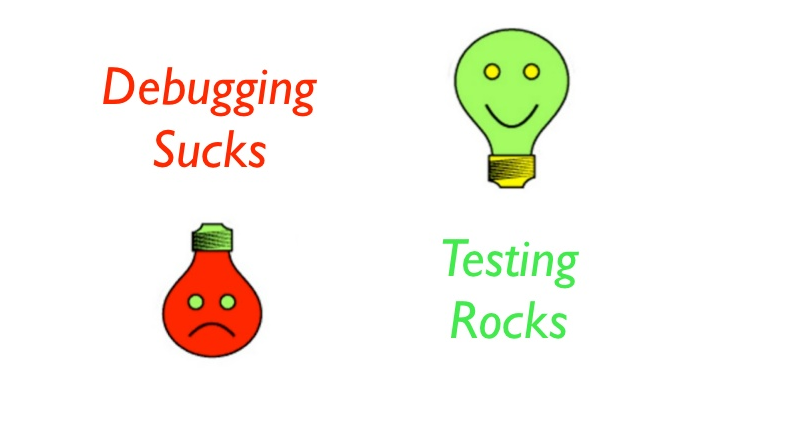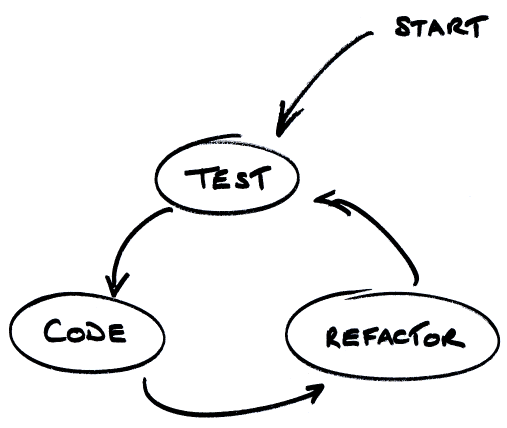One of our awesome full stack developers explains the benefits and the best methods of automated code testing, helping to create bug free products millions of people can enjoy.

What is it?

Automated code testing, as the name suggests, consists of automatically testing a software or a piece of code so that given some input data and some preconditions, the final result meets the expectations. In other words, you check that your code does what it was intended to do.

How is it done?Automated tests are also programs, so you also have to write code to make them. For example, let us suppose that we write a library: given a set of three points, it returns an object that represents a triangle (with some methods to calculate perimeter, area, height, etc). Now the question is: how can we test this library?

The simple way…

…is providing a set of different use cases (that is, different triangles) and for each of them make the calculations and check that the final result is the expected one. In this set corner-cases should always be considered. For example, for this library the case of giving the 3 same points, the case of providing 2 equal points and the case of provided 3 aligned points should be considered. The rest of the set could be completed with equilateral, isosceles, scalene and right triangles.

The tests could look like this in pseudo-code:

```Load set of test triangles
For each triangle in set
calculate perimeter
calculate area
calculate height
check results match expectations
```

That simple?

This is just the basics. The example is very simple because the tested library has no external dependencies. In some cases, the dependencies should be “mocked” so that we can gain control over them – enabling us to provide different conditions and check how the library reacts to all of them. This is done differently depending on the language, but the main concept remains the same.